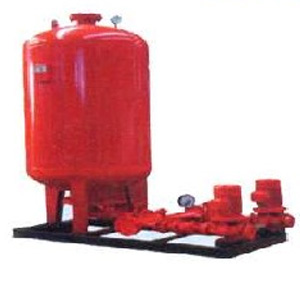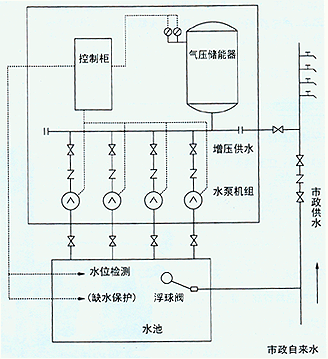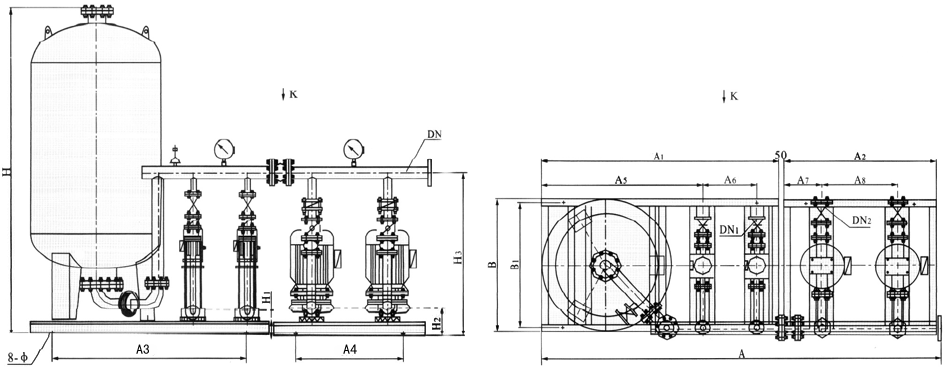﻿ XQ型消防稳压给水设备-型号-价格 - 永嘉龙洋泵阀有限公司

### 供水设备••••# XQ型消防稳压给水设备

### 1XQ型消防稳压给水设备适用范围

1、工业、民用建筑的消防稳压给水；
2、暖通、空调、锅炉的定压补水；
3、隐蔽工程、临时建筑和小型工矿，偏远地区的生活给水。

### 2XQ型消防稳压给水设备产品特点

1、设备体积小、结构紧凑、不受供水场合限制，可设置在任何高度向消防管网供水；
2、具有投资省、建设周期短、施工安装简便、便于实现自动控制等优点；

### 4XQ型消防稳压给水设备结构图### 5XQ型消防稳压给水设备型号参数

 序号 稳压设备型号 消防压力(Mpa)P1 隔膜式气压罐 配用水泵 运行压力 (Mpa) 型号规格 工作压力比αb 消防储水容积(L) 型 号 标定容积 稳压水容积 1 XQ G -Ⅰ-10 0.10 XQG600×0.6 0.60 150 159 ISG32-160 N=1.5kw P1=0.10 PS1=0.16 P2=0.13 PS2=0.31 2 XQ G -Ⅰ-16 0.16 XQG600×0.6 0.60 150 159 ISG32-200A N=2.2kw P1=0.16 PS1=0.26 P2=0.23 PS2=0.42 3 XQ G -Ⅰ-22 0.22 XQG600×0.6 0.60 150 159 ISG32-200 N=3.0kw P1=0.22 PS1=0.31 P2=0.28 PS2=0.46 4 XQ G(W) -Ⅰ-10 0.10 XQG800×0.6 0.60 300 320 ISG40-160 N=2.2kw P1=0.10 PS1=0.16 P2=0.13 PS2=0.31 5 XQ G(W) -Ⅰ-16 0.16 XQG800×0.6 0.60 300 320 ISG40-200A N=3.0kw P1=0.16 PS1=0.26 P2=0.23 PS2=0.42 6 XQ G(W) -Ⅰ-22 0.22 XQG800×0.6 0.60 300 320 ISG40-200 N=4.0kw P1=0.22 PS1=0.31 P2=0.28 PS2=0.46 7 XQ G(W) -Ⅱ-Z-38 0.22-0.38 XQW800×0.6 0.85 150 234 25LG3-10×6 N=2.2kw P1=0.38 PS1=0.48 P2=0.43 PS2=0.60 8 XQ G(W) -Ⅱ-Z-50 0.38-0.50 XQW800×1.0 0.85 150 234 25LG3-10×8 N=2.2kw P1=0.50 PS1=0.60 P2=0.56 PS2=0.75 9 XQ G(W) -Ⅱ-Z-65 0.50-0.65 XQW800×1.0 0.85 150 234 25LG3-10×10 N=3.0kw P1=0.65 PS1=0.81 P2=0.77 PS2=0.93 10 XQ G(W) -Ⅱ-Z-85 0.65-0.85 XQW800×1.6 0.85 150 234 25LG3-10×12 N=4.0kw P1=0.85 PS1=1.01 P2=0.93 PS2=1.10 11 XQ G(W) -Ⅱ-Z-100 0.85-1.00 XQW800×1.6 0.85 150 234 25LG3-10×14 N=4.0kw P1=1.00 PS1=1.16 P2=1.09 PS2=1.27 12 XQ G(W) -Ⅱ-X-38 0.22-0.38 XQG1000×0.6 0.80 300 302 25LG3-10×6 N=2.2kw P1=0.38 PS1=0.48 P2=0.43 PS2=0.60 13 XQ G(W)-Ⅱ-X-50 0.38-0.50 XQG1000×1.0 0.80 300 302 25LG3-10×8 N=2.2kw P1=0.50 PS1=0.60 P2=0.56 PS2=0.75 14 XQ G(W) -Ⅱ-X-65 0.50-0.65 XQG1000×1.0 0.80 300 302 25LG3-10×10 N=3.0kw P1=0.65 PS1=0.81 P2=0.77 PS2=0.93 15 XQ G(W) -Ⅱ-X-85 0.65-0.85 XQG1000×1.6 0.80 300 302 25LG3-10×12 N=4.0kw P1=0.85 PS1=1.01 P2=0.93 PS2=1.10 16 XQ G(W) -Ⅱ-X-100 0.85-1.0 XQG1000×1.6 0.80 300 302 25LG3-10×14 N=4.0kw P1=1.00 PS1=1.16 P2=1.09 PS2=1.27 17 XQ G(W) -Ⅱ-XZ-38 0.22-0.38 XQG1200×0.6 0.80 450 474 25LG3-10×6 N=2.2kw P1=0.38 PS1=0.48 P2=0.43 PS2=0.60 18 XQ G(W) -Ⅱ-XZ-50 0.38-0.50 XQG1200×1.0 0.80 450 474 25LG3-10×8 N=2.2kw P1=0.50 PS1=0.60 P2=0.56 PS2=0.75 19 XQ G(W) -Ⅱ-XZ-65 0.50-0.65 XQG1200×1.0 0.80 450 474 25LG3-10×10 N=3.0kw P1=0.65 PS1=0.81 P2=0.77 PS2=0.93 20 XQ G(W) -Ⅱ-XZ-85 0.65-0.85 XQG1200×1.6 0.80 450 474 25LG3-10×12 N=4.0kw P1=0.85 PS1=1.01 P2=0.93 PS2=1.10 21 XQ G(W) -Ⅱ-XZ-100 0.85-1.0 XQG1200×1.6 0.80 450 474 25LG3-10×14 N=4.0kw P1=1.00 PS1=1.16 P2=1.09 PS2=1.27

• 来自【河北省邢台市】客户反馈

消防稳压给水设备等单位工作人员验货通过才来评价，CCCF认证，质量过关，消防资质齐全，比较专业的一家

• 来自【黑龙江省哈尔滨市】客户反馈

一次在网上买消防设备，原本不敢买，只先买两台消火栓泵和一个配套控制箱，没想到安装上去效果真的很好，所以我陆陆续续把其他的设备都一起购买了，整个工程都已验收成功位

• 来自【广东省江门市】客户反馈

消防稳压设备厂家发货很快，以前买的用段时间就漏，老板介绍的这个磁力泵不使用机械密封，无泄漏点，使用效果更好，这次买的泵很满意，感谢老板的推荐

• 来自【内蒙古包头市】客户反馈

证书和AB证跟泵都收到了，消防网上可以查到，厂家很有责任心，物流提货全部都给安排好了，直接提货就行了产品包装完好，消防稳压给水设备已经上线使用，效果反应不错，考虑再买

• 来自【甘肃省金昌市】客户反馈

消防泵收到安装上去了 住5楼平常水很小 安装水泵后水流量很大 压力足